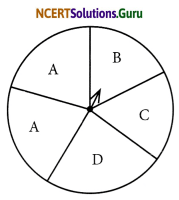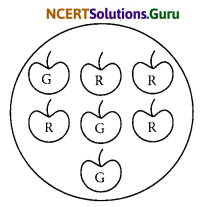# NCERT Solutions for Class 8 Maths Chapter 5 Data Handling Ex 5.3

These NCERT Solutions for Class 8 Maths Chapter 5 Data Handling Ex 5.3 Questions and Answers are prepared by our highly skilled subject experts.

## NCERT Solutions for Class 8 Maths Chapter 5 Data Handling Exercise 5.3

Question 1.
List the outcomes you can see in these experiments
(a) Spinning a wheel
(b) Tossing two coins together
Solution:
(a) Outcomes in spinning the given wheel are A, B, C, or D.
(b) Outcomes in tossing two coins together are HH, HT, TH, TT
[HT means Head on first coin + Tail on the second coin]Question 2.
When a die is thrown, list the outcomes of an event of getting.
(i) (a) prime number
(b) not a prime number
(ii) (a) a number greater than 5
(b) a number not greater than 5
Solution:
Possible outcomes are 1, 2, 3, 4, 5 and 6
(i) (a) Outcomes are 2, 3 and 5
(b) Outcomes are 1, 4 and 6
(ii) (a) Outcomes greater than 5 is 6
(b) Outcomes of getting a number not greater than 5 are 1, 2, 3, 4 and 5Question 3.
Find the
(a) Probability of the pointer stopping on D in (Question 1-(a))?
(b) Probability of getting an ace from a well-shuffled deck of 52 playing cards?
(c) Probability of getting a red apple. (See figure below)Solution:
(a) On the spinning wheel there are 5 sectors containing A, B, C, and D.
Since, there is only one sector containing D,
Number of possible outcome = 1
Number of equally likely outcomes = 5
∴ Probability = $$\frac{1}{5}$$

(b) Number of possible outcomes = 52
Since there are 4 aces in a pack of 52 cards, and out of the one ace can be obtained in 4 ways
∴ Probability of getting an ace = $$\frac{4}{52}=\frac{1}{13}$$

(c) There are 7 apples in all
Possible number of ways = 7
Since there are 4 red apples
No. of equally likely outcomes = 4
∴ Probability of getting a red apple = $$\frac{4}{7}$$Question 4.
Numbers 1 to 10 are written on ten separate slips (one number on one slip), kept in a box, and mixed well. One slip is chosen from the box without looking into it. What is the probability of?
(i) getting a number 6?
(ii) getting a number less than 6?
(iii) getting a number greater than 6?
(iv) getting a 1-digit number?
Solution:
Since there are 10 slips,
Total number of outcomes = 10
(i) We can get a slip containing the number ‘6’ only once
Number of favourable outcome = 1
∴ Probability of getting the number = $$\frac{6}{10}$$

(ii) Numbers less than 6 are 1, 2, 3, 4 and 5
No. of Favourable outcomes are 5
∴ Probability of getting a no. less than 6 = $$\frac{5}{10}=\frac{1}{2}$$

(iii) Numbers greaterthan 6 are 7, 8, 9 and 10
No. of favourable outcomes = 4
∴ Probability of getting a number greater than 6 = $$\frac{4}{10}=\frac{2}{5}$$

(iv) One-digit numbers are 1, 2, 3, 4, 5, 6, 7, 8 and 9
No. of favourable outcomes = 9
∴ Probability of getting a one-digit number = $$\frac{9}{10}$$Question 5.
If you have a spinning wheel with 3 green sectors, 1 blue sector, and 1 red sector, what is the probability of getting a green sector? What is the probability of getting a non-blue sector?
Solution:
There are 5 sectors is all (3 green + 1 blue + 1 red)
Total possible outcomes = 5
Since there are 3 green sectors
Number of favourable outcomes = 3
∴ Probability of getting a green sector = $$\frac{3}{5}$$
Again there are 4 non-blue sectors.
Number of favourable outcomes = 4
∴ Probability of getting a non blue sector = $$\frac{4}{5}$$

Question 6.
Find the probabilities of the events given in question 2.
Solution:
When a die is thrown there are 6 outcomes in all (1, 2, 3, 4, 5 and 6)
(i) Since there are 3 prime numbers 2, 3 and 5
Number of favourable outcomes = 3
∴ Probability of getting a prime number = $$\frac{3}{6}=\frac{1}{2}$$

(ii) Since there are 3 non-prime numbers (1, 4 and 6)
Number of favourable outcomes = 3
∴ Probability of getting a non prime number = $$\frac{3}{6}=\frac{1}{2}$$(iii) Since there is 1 number greater than 5 (i.e., 6)
Number of favourable outcome = 1
∴ Probability of number greater than 5 = $$\frac{1}{6}$$

(iv) Since there are 5 numbers which are not greater than 5 (i.e., 1, 2, 3, 4 and 5)
Number of favourable outcomes = 5
∴ The probability of a number which is not greater than 5 = $$\frac{5}{6}$$

error: Content is protected !!